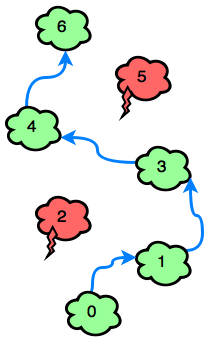# Jumping on the Clouds Hackerrank problem solution

##### Jumping on the Clouds Hackerrank

Emma is playing a new mobile game involving n clouds numbered from 0 to n-1. A player initially starts out on cloud c0, and they must jump to cloud cn-1. In each step, she can jump from any cloud i to cloud i+1 or cloud i+2.

There are two types of clouds, ordinary clouds and thunderclouds. The game ends if Emma jumps onto a thundercloud, but if she reaches the last cloud (i.e., cn-1), she wins the game!Can you find the minimum number of jumps Emma must make to win the game? It is guaranteed that clouds c0 and cn-1 are ordinary-clouds and it is always possible to win the game.

Input Format

The first line contains an integer, n (the total number of clouds).
The second line contains n space-separated binary integers describing clouds c0, c1,….,cn-1.

If ci=0, the ith cloud is an ordinary cloud.
If ci=1, the ith cloud is a thundercloud.
Constraints
2<=n<=100 c{0,1} c0=cn-1=0 Output Format Print the minimum number of jumps needed to win the game. Sample Input 0 7 0 0 1 0 0 1 0 Sample Output 0 4 Sample Input 1 6 0 0 0 0 1 0 Sample Output 1 3 Explanation Sample Case 0: Because c2 and c5 in our input are both 1, Emma must avoid c2 and c5. Bearing this in mind, she can win the game with a minimum of 4 jumps:Sample Case 1:
The only thundercloud to avoid is c4. Emma can win the game in 3 jumps:##### Solution in Java
``````import java.io.*;
import java.util.*;
import java.text.*;
import java.math.*;
import java.util.regex.*;

public class Solution {

public static void main(String[] args) {
Scanner in = new Scanner(System.in);
int n = in.nextInt();
int c[] = new int[n];
int zero = 0;
int jump = 0;
for(int c_i=0; c_i < n; c_i++){
c[c_i] = in.nextInt();
if(c[c_i]==1) {
jump += zero/2+1;
zero = 0;
}
else
zero ++;
}
jump += zero/2;
System.out.print(jump);

}
}``````

python

``````#!/bin/python3

import sys

n = int(input().strip())
c = [int(c_temp) for c_temp in input().strip().split(' ')]

res = 0
i = 0
while i < n-1:
if i+2<n and c[i+2] == 0:
i = i+2
res += 1
else:
i = i+1
res += 1
print(res)``````
##### C++
```#include <map>
#include <set>
#include <list>
#include <cmath>
#include <ctime>
#include <deque>
#include <queue>
#include <stack>
#include <string>
#include <bitset>
#include <cstdio>
#include <limits>
#include <vector>
#include <climits>
#include <cstring>
#include <cstdlib>
#include <fstream>
#include <numeric>
#include <sstream>
#include <iostream>
#include <algorithm>
#include <unordered_map>

using namespace std;

int main(){
int n;
cin >> n;
vector<int> c(n);
for(int c_i = 0;c_i < n;c_i++){
cin >> c[c_i];
}
vector<int>d(n, 10000);
d = 0;
for (int i = 0; i < n; ++i) {
if (c[i] == 1) continue;
if (i + 1 < n && c[i + 1] == 0) {
d[i + 1] = min(d[i + 1], d[i] + 1);
}
if (i + 2 < n && c[i + 2] == 0) {
d[i + 2] = min(d[i + 2], d[i] + 1);
}
}
cout << d[n - 1] << endl;
return 0;
}
```

### hasectic

A web developer(Front end and Back end), and DBA at csdamu.com. Currently working as Salesforce Developer @ Tech Matrix IT Consulting Private Limited. Check me @about.me/s.saifi